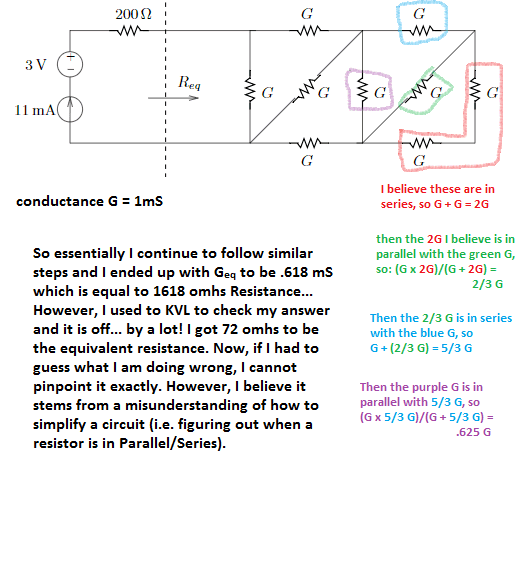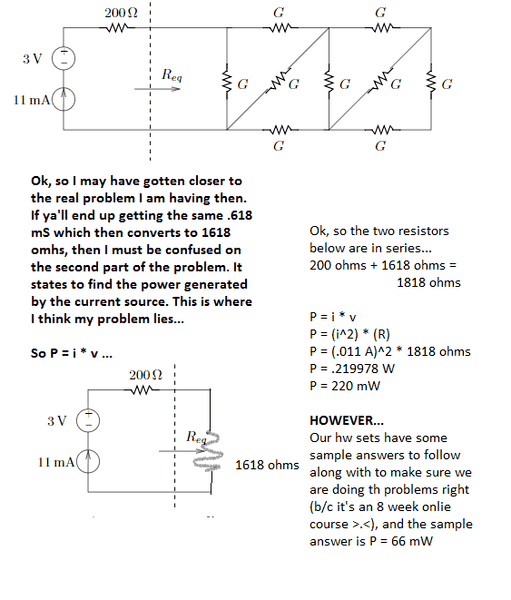# Simplifying a Circuit w/ Parallel Resistors

• Engineering
• YellowBelliedMarmot

#### YellowBelliedMarmot

Member advised to use the homework template for posts in the homework sections of PF.
The goal of this problem is to find the equivalent resistance of all the resistors. Each resistor shown in the image has a conductance of 1 mS which I believe is equal to 1000 ohms. I attached a picture of the circuit diagram in which I show some of my work in order to convey my erroneous thought process. I tried color-coating everything to make it more readable. Thanks in advance! :{DA good way to approach this is to rearrange the diagram so that current only flows from left to right or vertically, never right to left (which includes diagonals that flow right & down or right & up).

Start by labeling all six junctions in the original diagram as A,B,...,F, starting at top left and going around clockwise. Then start a new left-to-right (l2r) diagram by putting A (top-left junction on initial diagram) at the far left and F (bottom-left junction in original) at the far right. Remember that shape and geometry are irrelevant. Only connections and resistances between connections matter.

Now work through the circuit, one junction at a time, transposing it to your l2r diagram. At each junction, say it is an n-way junction and you have m wires entering it from the left. n will be at least 3, otherwise it is not a junction. Then draw n-m wires going out to the right. For each wire, if the downstream junction is already drawn on the l2r diagram, connect it to that, approaching from the left (redraw the diagram if necessary, lengthening some wires, to make that possible). Otherwise, mark the downstream junction on your l2r diagram and draw the connection to that, again approaching it from the left.

Do this until all wires and junctions have been marked on the l2r diagram.

Then check that , in the l2r diagram:
1. the number of wires at each of your junctions matches that on the original diagram; and
2. The resistors on each wire between junctions match those on the original diagram.

Make corrections as necessary, based on any errors revealed by the check.

You now have the circuit drawn in a fashion in which it is straightforward to work out resistances. Use the parallel and series laws repeatedly to collapse the diagram until there remains only one equivalent wire between A and F. The resistance of that is the resistance of your circuit.

Good luck!

PS I get the same answer as you. ##0.6\dot1\dot8## mS. Perhaps there is an error in your KVL check. Can you post your work on that?

Last edited:
The goal of this problem is to find the equivalent resistance of all the resistors. Each resistor shown in the image has a conductance of 1 mS which I believe is equal to 1000 ohms. I attached a picture of the circuit diagram in which I show some of my work in order to convey my erroneous thought process. I tried color-coating everything to make it more readable. Thanks in advance! :{DView attachment 100846
That all looks correct to me. You should be able to finish it by continuing that way.

I think the reason the result doesn't agree with the naïve KVL calc (for which I also get 72 Ohms) is that that calc does not take account of the voltage across the terminals of the current source, which will be large - it increases with the resistance of the load. So KVL cannot be used to check the resistance calc in the presence of a current source, because the voltage across the current source is whatever it has to be to make the KVL law hold.

If you want to use KVL to check the resistance calc, first get rid of the current source. Then it should work.*online ;D

One thing to note is that conductances in series do not add as a simple sum (arithmetically). Conductances in series add in the way that resistances in parallel add. That is, if two conductances G1 and G2 are in series then the total conductance is given by G1*G2/(G1 + G2).

•FactChecker
Req is not 1618 ohms.

•CWatters
I am not getting the same numbers as you, but I agree up to and including the blue step of the OP with (5/3)G .

At that point you can simplify, because on the left you have a circuit exactly the same as the one you have already collapsed, so you don't have to think about calculating it - you finish with two conductances of (5/3)G and one of G all in parallel - total conductance (13/3)G I think?

Added: if that's right and R is 1,000 Ω then the equivalent resistance of that part of the circuit would be
3000/13 ≈ 231 Ω , which is then in series with another 200 Ω .

Last edited:
Req is not 1618 ohms.

+1

Req must be less than 1000 Ohms because of the resistor immediately to the right of the word "Req" on the diagram.

You can also deduce that Req must be greater than 500 Ohms by inspecting the circuit.